# ResNet-50 Trained onImageNet Competition Data

Identify the main object in an image

Released in 2015 by Microsoft Research Asia, the ResNet architecture (with its three realizations ResNet-50, ResNet-101 and ResNet-152) obtained very successful results in the ImageNet and MS-COCO competition. The core idea exploited in these models, residual connections, is found to greatly improve gradient flow, thus allowing training of much deeper models with tens or even hundreds of layers. ImageNet classes are mapped to Wolfram Language Entities through their unique WordNet IDs.

Number of layers: 177 | Parameter count: 25,610,216 | Trained size: 104 MB

## Examples

### Resource retrieval

Get the pre-trained net:

 In:=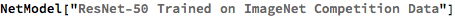Out=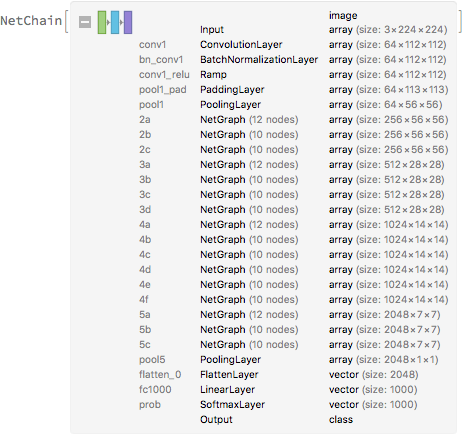### Basic usage

Classify an image:

 In:=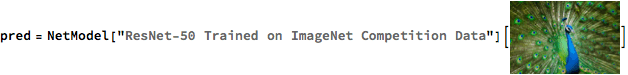Out=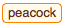The prediction is an Entity object, which can be queried:

 In:=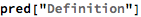Out=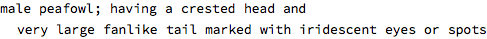Get a list of available properties of the predicted Entity:

 In:=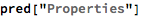Out=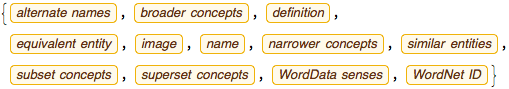Obtain the probabilities of the ten most likely entities predicted by the net:

 In:=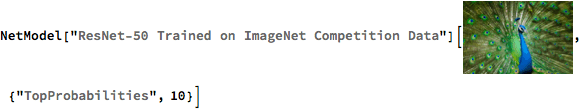Out=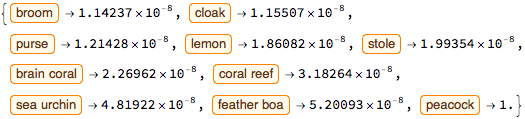An object outside the list of the ImageNet classes will be misidentified:

 In:=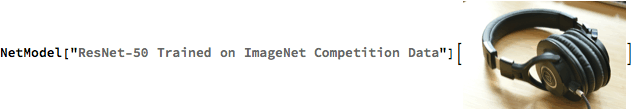Out=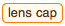Obtain the list of names of all available classes:

 In:=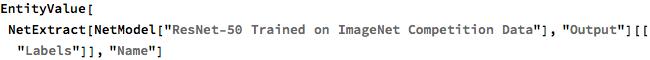Out=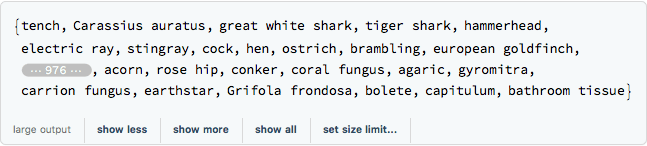### Feature extraction

Remove the last three layers of the trained net so that the net produces a vector representation of an image:

 In:=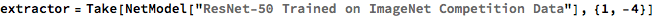Out=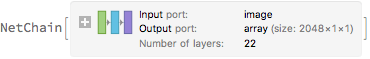Get a set of images:

 In:=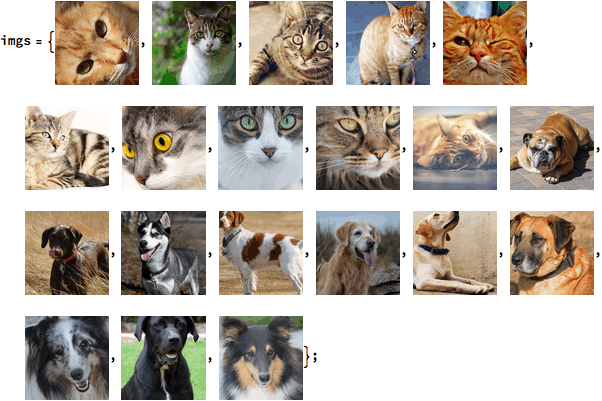Visualize the features of a set of images:

 In:=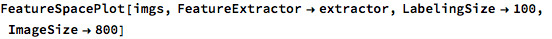Out=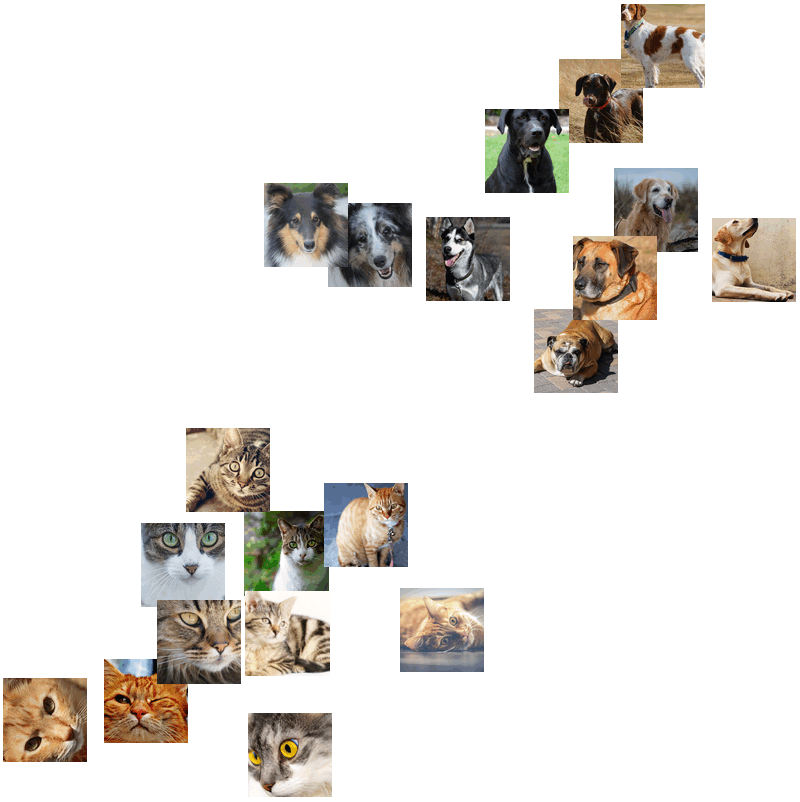### Visualize convolutional weights

Extract the weights of the first convolutional layer in the trained net:

 In:=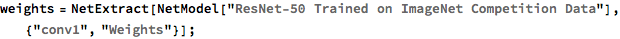Visualize the weights as a list of 64 images of size 7x7:

 In:=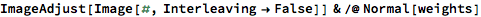Out=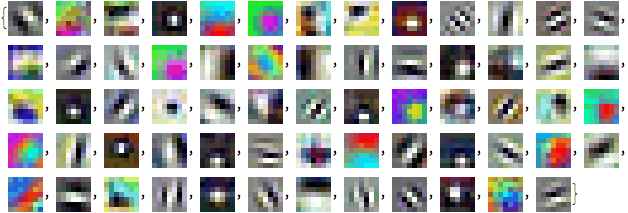### Transfer learning

Use the pre-trained model to build a classifier for telling apart images of dogs and cats. Create a test set and a training set:

 In:=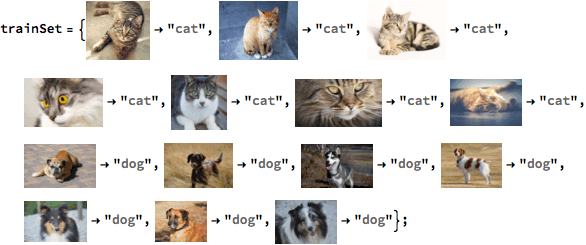In:=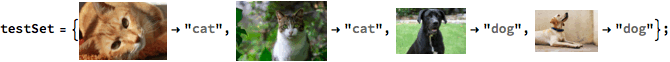Remove the linear layer from the pre-trained net:

 In:=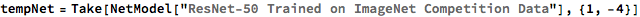Out=Create a new net composed of the pre-trained net followed by a linear layer and a softmax layer:

 In:=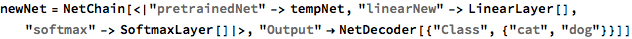Out=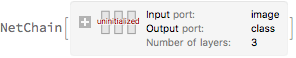Train on the dataset, freezing all the weights except for those in the "linearNew" layer (use TargetDevice -> "GPU" for training on a GPU):

 In:=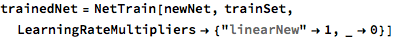Out=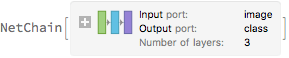Perfect accuracy is obtained on the test set:

 In:=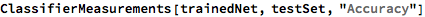Out=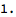### Net information

Inspect the number of parameters of all arrays in the net:

 In:=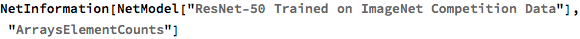Out=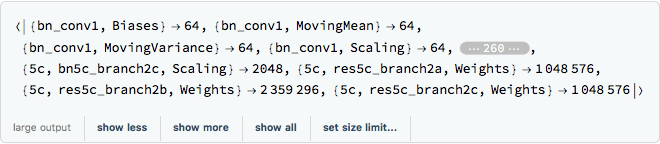Obtain the total number of parameters:

 In:=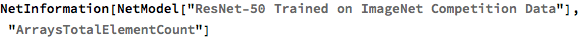Out=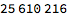Obtain the layer type counts:

 In:=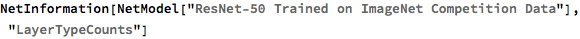Out=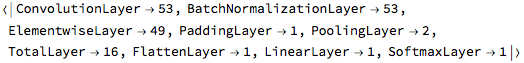Display the summary graphic:

 In:=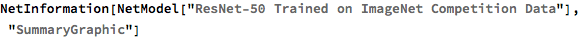Out=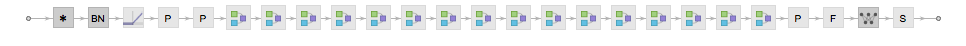### Export to MXNet

Export the net into a format that can be opened in MXNet:

 In:=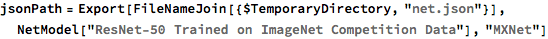Out=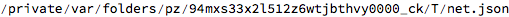Export also creates a net.params file containing parameters:

 In:=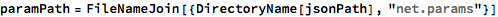Out=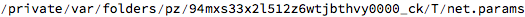Get the size of the parameter file:

 In:=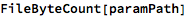Out=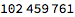The size is similar to the byte count of the resource object:

 In:=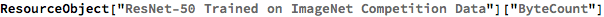Out=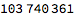## Requirements

Wolfram Language 11.2 (September 2017) or above